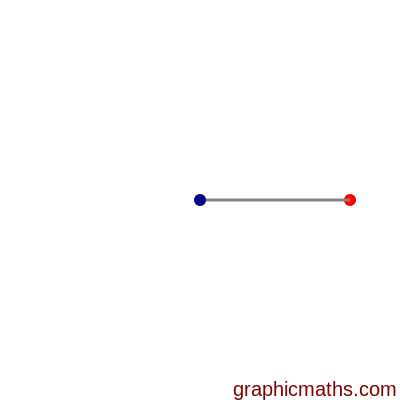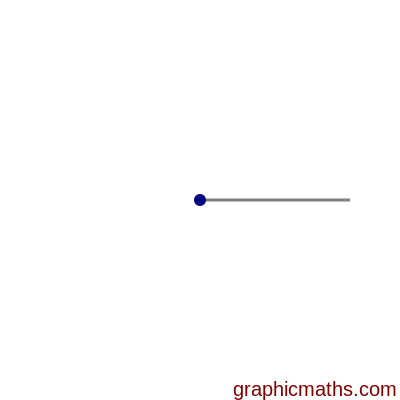# Locus of points a fixed distance from a given point

Martin McBride, 2020-08-22
Tags locus
Categories maths gcse geometry

The locus of all points that are a fixed distance r from a given point P is a circle. The circle has a radius r and has its centre at P.

In this animation, the blue dot represents P, and the grey line represents the distance r. The circle is formed as the red dot moves through all the points in the locus, tracing out the circle:## Points that are less than a fixed distance from a given point

The points that are less than a fixed distance r from the point P are all the points that fall inside the circle. This is illustrated below:This topic is also covered by this video: